American Journal of Theoretical and Applied Statistics
Volume 4, Issue 3, May 2015, Pages: 112-117

Statistical Trend Analysis of Residential Water Demand in Kisumu City, Kenya

Robert Nyamao Nyabwanga1, *, Edgar Ouko Otumba2, Fredrick Onyango2, Simeyo Otieno3

1Department of Mathematics, Kisii University, Kisii, Kenya

2Department of Statistics and Actuarial Science, Maseno University, Maseno, Kenya

3School of Business and Economics, Jaramogi Oginga Odinga University, Bondo, Kenya(R. N. Nyabwanga)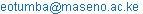(E. O. Otumba)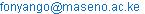(F. Onyango)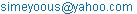(S. Otieno)

Robert Nyamao Nyabwanga, Edgar Ouko Otumba, Fredrick Onyango, Simeyo Otieno. Statistical Trend Analysis of Residential Water Demand in Kisumu City, Kenya. American Journal of Theoretical and Applied Statistics. Vol. 4, No. 3, 2015, pp. 112-117. doi: 10.11648/j.ajtas.20150403.16

Abstract: This study sought to analyse trend in the monthly water demand data series in Kisumu city at both seasonal and non-seasonal levels using the parametric method of Ordinary Least Squares (OLS) and non-parametric methods of Mann-Kendall tau and Sen's T test.  Sen’s test was applied to validate the Mann Kendall trend test and to estimate the magnitude of the trend and its direction. The significance of the slope of the OLS equation was tested using the F-Test based on the Analysis of Variance (ANOVA). Secondary monthly water consumption data obtained from KIWASCO for the period January 2004 to December 2013 were used. Using logarithmically transformed data, the study established by OLS that residential water demand in Kisumu City had a significant increasing trend (FCalc=(105.13) > F(1;119)(α=0:05)=(5.15)). Kendall's tau test corroborated the OLS results of a significant increasing trend. The Sens T test indicated that most of the months registered significant upward trend with Sen’s slope estimates showing positive rates of change in residential water demand for these months.

Keywords: Trend, Kendall’s Tau, OLS, Sen’s T, Residential Water Demand

1. Introduction

World Bank Report of 2005 observes that poor access to water supply is often a result of poor policies and management practices. However, there is significant disagreement over the approach to addressing the problem. Likewise in the World Bank Report of 2003  it is argued that a first and crucial step towards improving water situation and its management is to treat water as an economic good. The Economist of July 19-25, 2003, argues that the problem above all, is that it has been colossally underpriced and that to meet the target of halving the proportion of people without access to clean water money will play a part.

Water is therefore viewed as an economic as well as social good. But greater reliance on pricing and markets are even more crucial. Council of Kisumu under the Companies Act Chapter 486 of the Laws of Kenya, established a water company by the name Kisumu water and Sewerage Company (KIWASCO) in 2001 but became operational in 2003. KIWASCOs mandate is to effectively and efficiently provide adequate water to customers and collect, treat and dispose sewerage in a safe and environment friendly manner .

In Kisumu City, the mean household water consumption is 149.50 l per day, resulting in a mean per capita of 32.92 L per day. According to a study by , the daily per capita water use in Kenya is 45.2 L. using the recommended basic water requirement of 50 l/c/d by , in the study area, there is a mean daily water per capita shortfall of 17.18 L. Wagah et al.further assert that only 25 percent of the households access the minimum recommended basic water requirement of 50 l/c/d.

Kisumu City residents obtain water from individual connections, yard tap connections, public tap connections, boreholes, springs and water vendors. As of September 2008, KIWASCO had 7,704 domestic water connections and 287 water kiosks . About 52 percent of Kisumu residents used piped water delivered to dwellings or compounds, and 13 percent depended on protected shallow wells/springs or roof catchment . Hence 65 percent of Kisumu residents had access to an improved water source, while 35 percent relied on unimproved water sources, including water vendors, open wells/springs, streams and ponds . However, Most residents in informal settlements only have access to water of poor quality, mainly because their water often comes from shallow wells.

Statement of the Problem

The rate of population growth, migration for work, long periods of warm years and the dust factor have over the years caused an increase in water demand in Kisumu City leading the current net demand of 27000 m3/day. The Kisumu Water Supply System, managed by KIWASCO, has a current net capacity of 5400 m3/day which is far much below the demand. For the City's water supply system to efficiently bridge the gap between water supply and water demand there is need to understand the general trend of the City's water demand so as to provide effective water demand management and fairly accurate future forecasts based on the trend.

2. Materials and Methods

There are several methods and tools for detecting trends and breaks in the hydrological series . However, the choice of any particular method must ensure accurate highlight of all the events related to variability and change and their impact on future prediction .

2.1. Description of Ordinary Least Square Models

Two kinds of trend models were developed to study the residential water demand trend problem in Kisumu City.

The first was the OLS linear trend equation given as: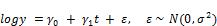(2.1)

Where, y is the dependent or response variable representing the amount of water demanded monthly, t is the covariate or explanatory variable andis the unobserved error or disturbance. The goal will be to estimate the regression parameters the intercept i.e.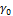and the slope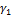. The least squares estimate of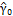and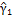are obtained using the following equations: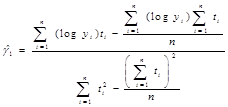(2.2)

And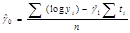(2.3)

The hypothesis tested was: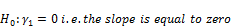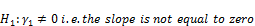The test statistic used was the F-Test based on the Analysis of Variance (ANOVA) which is summarized in the table1 below:

Table 1. ANOVA table.

 Source of variation df SS MS F-ratio Regression 1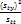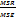Error n-2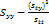Total n-1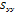Where;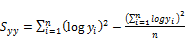(2.4)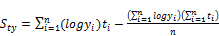(2.5)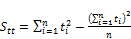(2.6)

The decision will be to reject H0 if the calculated F value is greater than F (1, n-1) (α=0.05)

As asserted by Gupta , the line obtained by OLS is the line of best fit because it is the line from where the sum of positive and negative deviations is zero i.e.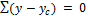and the sum of the squares of the deviations i.e.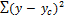is least.

The second trend model was the quadratic trend equation of the form: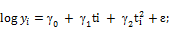(2.7)

Where,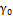is the intercept;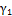is the linear term;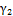is the quadratic term; andis the residual.

2.2. Mann-Kendall's Tau Test of Trend

According to Onoz and Bayazit , the Kendall'sstatistic is one of the non-parametric trend tests that have been frequently used and is considered an excellent reference for numerous other trend test techniques. Kendal'stest first ranks all observations by date order, then the difference between each consecutive value is calculated and the sum of the signs of these differences is calculated as the Kendall sum, S statistic given as in the Equation below: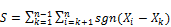(2.8)

The application of trend test is done to a time series Xk that is ranked from k = 1,2,…, n-1 and Xi, which is ranked from I = k+1, 2,.., n. Each of the data point xi is taken as a reference point which is compared with the rest of the data points Xi so that;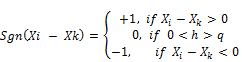(2.9)

When n ≥ 8, the statistic S is approximately normally distributed with the mean. E(S) =0. The test statistics Zc is computed as follows: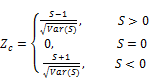(2.10)

Zc here follows a standard normal distribution. The variance statistic is given as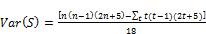(2.11)

t indicates the extent of any given time and the summation is across all ties in the water demand data.

Under the Kendall'stest, a positive value of S in Equation (3.4) indicates an increasing trend whereas a negative value indicates a decreasing trend . The null and alternative hypotheses under S are:

H0: There is no trend in the monthly demand for water.

H1: There is a trend in the monthly demand for water.

The decision was to reject the null hypothesis if the p-value of the test is less than the level of significance. Kendall'stest of significance will be two-sided and considered significant at the 0.05 level.

Test for serial autocorrelation will be done and if this will be detected, the time series should be ‘pre-whitened’ to eliminate the effect of serial correlation before applying the Mann–Kendall test . This will be implemented using the following procedure: If we let X1, X2…..Xn be residential water observations at times 1, 2,..…n measured in months, then:

1. First, we compute the lag-1 serial correlation coefficient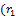)

2. If the calculated) is not significant at the 5% level, then the Mann–Kendall test will be applied to original values of  the time series.

3. However, if the calculated) is significant, prior to application of the Mann–Kendall test, then the pre-whitened time series may be obtained as: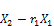,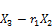,……,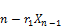2.3. Sen’s T Test

This is an aligned rank technique that first removes the seasonal effect from each datum, then sums the data over seasons, and finally produce a statistic from these sums. It is more powerful than its counterpart. It is distribution free and not affected by seasonal fluctuations . The computational steps are as follows:

i.        Compute the average for the month i,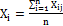and the average for the year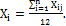Subtract the monthly average from each of the corresponding months in the n years to remove seasonal effects.

ii.        Rank all the differences from 1 to number of months times the number of years to obtain the matrix (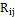) where,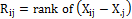among the 12n values of differences.

iii.        The ranks for each year are averaged  i.e.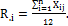Also, the rank for each monthiv.        Calculate the test statistic;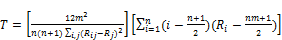(2.12)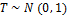under the null hypothesis of no trend. Positive values of T indicate an upward trend and negative values indicate negative trend. The decision was to reject the hypotheses of no trend if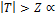exceeds a prespecified percentile of the normal distribution, where Z is the standard normal variate.

2.4. Sen’s Slope Estimator

If a linear trend is present in a time series, then the true slope (change per unit time) can be estimated by using a simple non-parametric procedure developed by Sen (1968). The magnitude of trend is predicted by the Sen’s estimator.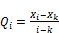(2.13)

where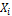and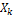are corresponding data values at time i and k and i >k. The median of the N values of Ti is represented as Sen’s estimator of slope which is given as: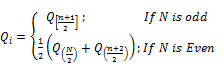(2.14)

Positive value of Qi indicates an increasing trend whereas a negative value indicates a decreasing trend in the residential water demand time series data.

Table 2. Descriptive Statistics.

 Descriptive Statistics Significance Test 5.00% Target P-Value SIG? AVERAGE: 93259.8 0.000 0.00% TRUE STD DEV: 23159.64 SKEW: 0.88 0.000 0.01% TRUE EXCESS-KURT: -0.62 0.000 6.28% FALSE MEDIAN: 83225 MIN: 64270 MAX: 147469 Q 1: 75863.5 Q 3: 113622

3. Results and Discussions

3.1. Descriptive Statistics

The Mean residential water demand was 93259.8M3 and is significantly different from zero. The Density distribution of residential water demand is significantly positively skewed and the density do not have fat tails. Half of the observations fall between 75863.5 and 113622 which imply that the quartiles (Q1, Q3) inscribe 50% of the values in the sample.

The skewness index of 0.88 indicated that the data exhibited positive skewness hence not normally distributed. Logarithm transformed values were used in further analysis.

3.2. OLS Estimation of Linear Trend for Residential Water Demand Data

The least squares estimates ofandare: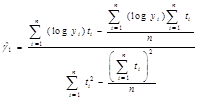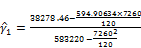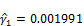And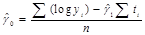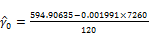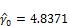Therefore the OLS trend equation is:(3.1)

To test the significance of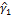, the F-test based on ANOVA was used.

i.        The hypotheses tested were:

H0: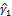= 0 i.e. the slope is equal to zero

H1: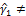0 i.e. the slope is not equal to zero.

ii.        Level of Signicance : α = 0:05

The results of the test are illustrated in an ANOVA table 3 below

Table 3. ANOVA Table.

 Source of Variation df SS MS F-Ratio Regression 1 0.570545 0.570545 105.13 Error 118 0.640418 0.005427 Total 119 1.210963

Fcritical = F(1;119)( α/2=0:025) = 5.15

Since 105.13 > 5.15, the null hypothesis is rejected and the alternative hypothesis that the slope is not equal to zero is accepted at 0.05 level of significance. Based on the OLS procedure, it is therefore concluded that there is a significant positive trend in residential water demand in Kisumu City as indicated by the positive= 0.001991.

Graphically, the Linear trend plot of the residential water demand in Kisumu City is illustrated in figure 1 below: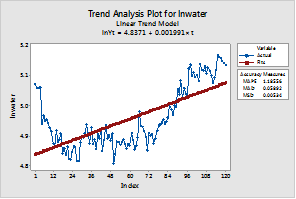Figure 1. Residential water demand linear trend plot.

3.3. Ordinary Least Squares Estimation of Quadratic Trend for Residential Water Demand Data

The quadratic trend equation was of the form:(3.2)

in which,is the intercept;is the linear term;is the quadratic term; andis the residual.

The parameter estimates for the quadratic trend model are shown in the table 4 below:

Table 4. Quadratic Trend Parameter Estimates.

 Parameter Parameter Estimates SE P-Value4.9766 0.011 <0.001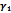-0.004867 0.000 <0.001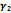-0.000057 0.000 <0.001

The OLS results as shown in Table 4 indicate that both the linear and quadratic terms were significantly different from zero at the 0.05 level. Therefore, the quadratic trend equation is: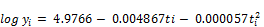(3.3)

From the results it can be noted that a change in both the sign and magnitude of the linear term. Inclusion of a quadratic term in the model changed the sign of the linear term from positive to negative. Moreover, the magnitude of the estimate of the termchanged from 0.001991 to -0.004867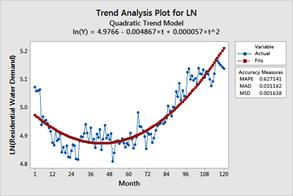Figure 2. Residential water demand quadratic trend plot.

3.4. Comparison Between the Linear Trend and Quadratic Trend

To determine which trend model best fits the residential water consumption for Kisumu City, the mean absolute percentage error (MAPE), Mean Absolute deviation (MAD) and Mean Square Deviation (MSD). The results of the analysis are shown in the table 5 below:

Table 5. Model Accuracy Measures.

 Trend Model MAPE MAD MSD Linear Trend 1.18556 0.05892 0.00534 Quadratic Trend 0.627141 0.031162 0.001638

The results of the analysis show that the quadratic trend equation had lower MAPE, MAD and MSD values implying that the quadratic trend equation fits the data well compared to the linear trend.

3.5. Mann-Kendall's Tau Test

Under the Kendall's tau test for trend the hypotheses to be tested were;

H0: There is no trend in the monthly demand for water.

H1: There is a trend in the monthly demand for water.

Table 6. Mann-Kendall trend test / Two-tailed test.

 Statistic Estimate Kendall’s Tau 0.482 S 3440.000 Var(S’) 194366.667 p-value(two-tailed) <0.0001 Alpha 0.05

As the computed p-value is lower than the significance level alpha=0.05, we should reject the null hypothesis H0 that there is no trend in the monthly demand for water in Kisumu City, and accept the alternative hypothesis H1 that there is trend in the monthly demand for water in Kisumu City. These results corroborate the OLS results obtained earlier.

3.6. Kendall's Tau Test for Seasonal Trend

In this test, we consider the fact that the time series are seasonal with a seasonality of 12 months. The seasonal Mann-Kendall test takes into account the 12 month seasonality and tests whether there is a trend due to seasonality. The hypotheses tested were:

H0: There is no seasonal trend in the water demand series

H1: There is a seasonal trend in the water demand series

The results of the test are as illustrated in table 7 below:

Table 7. Seasonal Mann-Kendall Test / Period = 12 / Serial independence / Two-tailed test (water demand).

 Parameter Estimate Kendall’s tau 0.530 S’ 286.000 P-value <0.0001 Alpha 0.05

As the computed p-value is lower than the significance level alpha=0.05, we reject the null hypothesis H0 that there is no seasonal trend in the monthly demand for water in Kisumu City, and accept the alternative hypothesis H1 that there is seasonal trend in the monthly demand for water in Kisumu City. The Kendall's tau is larger when we take into account the seasonality as compared to when seasonality is not taken into account. We therefore conclude that there is a trend in the water demand time series when we take into account the seasonality.

3.7. Sen’s T Test for Within Month’s Trend

From table 8 we observe that the months of January, June, July, August, September, October, November and December have significant trends at α=5%. The months of February, March, April and May show insignificant trend at 5% level of significance. Based on the result we conclude that most of the months of Kisumu City residential water consumption show significant trend. According to Sen's T test all months show upward trend. The slope estimates show positive rates of change for these months.

Table 8. Residential Water Demand in Kisumu City.

 Mann-Kendall trend Sen's slope estimate Time series First year Last Year n Test Z Signific. Q Qmin95 Qmax95 January 2004 2013 10 2.33 * 3153.500 573.177 9968.862 February 2004 2013 10 1.07 3335.000 -3547.679 11372.046 March 2004 2013 10 1.25 2833.000 -1574.440 10320.110 April 2004 2013 10 1.25 3832.833 -2145.302 9576.342 May 2004 2013 10 1.79 + 3405.000 -637.871 8648.419 June 2004 2013 10 2.50 * 6918.400 1727.468 8947.539 July 2004 2013 10 2.33 * 6146.800 1809.165 11525.671 August 2004 2013 10 2.50 * 7683.000 2147.108 12093.054 September 2004 2013 10 2.15 * 6634.500 461.944 12004.105 October 2004 2013 10 2.33 * 7545.000 1129.759 13453.994 November 2004 2013 10 2.15 * 6852.800 1160.380 12179.088 December 2004 2013 10 2.86 ** 7145.500 2466.940 11958.556 ANNUAL 2004 2013 10 2.50 * 4808.083 1242.168 10520.311

** trend at α = 0.01 level of significance; * trend at α = 0.05 level of significance; + trend at α = 0.1 level of significance

4. Conclusions

In this study we statistically analyzed the trend in residential water demand data in Kisumu City. The OLS and Kendall’s tau Tests both revealed positive significant trend. Sen’s Slope estimators also indicated increasing magnitude of slope in correspondence with the Mann-Kendall Test values. Majority of the months show upward significant trend while some of the months showed trend which was however not significant.

The study further established a larger Kendall's tau when seasonality was taken into account as compared to when seasonality was not taken into account. We therefore conclude that there is monthly seasonal trend in the residential water demand for Kisumu City.  The Sen's T test showed that slope estimates showing positive rates of change. Further study of trend may reveal other trend aspects which will be helpful in taking necessary action for water management activities in Kisumu City.

Acknowledgment

We thank Kisumu Water and Sewerage Company Limited and more especially Frank David Ochieng the Ag.Head of Commercial Services for the provision of the residential water use data.

References

1. Gupta, S., P.(2005). 'Statistical Methods, Sultan Chand and Sons Educational Publishers, New Delhi.
2. Kahya, E. and S. Kalayci, 2004. Trend analysis of stream flow in Turkey. J. Hydrol., 289: 128-144.DOI:10.1016/j.jhydrol.2003.11.006.
3. KIWASCO (2007). Kisumu Water and Sewerage Company Strategic Plan 2007-2012.
4. Machiwal D, Jha MK.(2008). Comperative evaluation of statistical tests for time series analysis: Application to hydrological timeseries. Hydrological Sciences, Journal-des Sciences Hydrologiques] 53(3): 353–366
5. Maftei C., Barbulescu, A. (2008). Statistical Analysis of Climate Evolution in Dobrudja Region. Proceedings of the World Congress on Engineering 2008 Vol II WCE 2008, July 2-4, London, U.K.
6. Onoz, B. and Bayazit, M. (2003). The power of statistical tests for trend detection.
7. Sen PK (1968). Estimates of the regression coefficient based on Kendall’s tau. J. Am. Stat. Assoc. 39:1379–1389
8. Wagah, G., Onyango, G. and Kibwage, J. (2010). Accessibility of water services in Kisumu Municipality Kenya. Journal of Geography and Regional Planning. Vol. 3(4), pp 114-125.
9. World Bank (2003) Water Resources Strategy. World Bank, Washington, D.C.
10. World Bank (2005) Water for the Urban Poor: Water Markets, Household Demand, and Service Preferences in Kenya. Water Supply and Sanitation Sector Board p.
11. Von Storch, H., (1995). Misuses of statistical analysis in climate research, in Analysis of Climate Variability: Applications of Statistical Techniques, edited by H. V. Storch and A. Navarra, pp. 11-26, Springer-Verlag, NewYork.

 Contents 1. 2. 2.1. 2.2. 2.3. 2.4. 3. 3.1. 3.2. 3.3. 3.4. 3.5. 3.6. 3.7. 4.
Article ToolsAbstractPDF(315K)